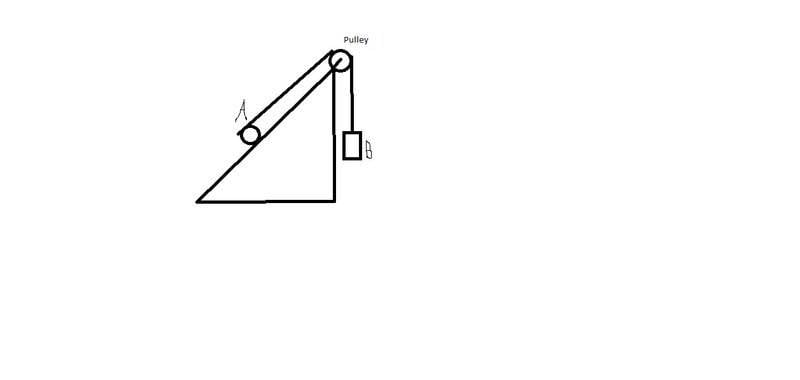# Total acceleration?

## Homework Statement,
the pulley, object a and object b all have the same mass
The cylinder rotates without sliding

## The Attempt at a Solution

Okay, so my teacher said that the frictional force oppose the line tension,
Object 1(cylinder)
$$\sum torque=(line tension2 . radius) - (frictionalforce . radius) = m.a$$
Pulley
Object B
Line tension1 - weight = m . a
what I don't get is why my teacher said that object a's acceleration is the total of its tangential acceleration and its linear acceleration.

Last edited:

Doc Al
Mentor
what I don't get is why my teacher said that object a's acceleration is the total of its tangential acceleration and its linear acceleration.
You need to relate the linear acceleration of object A to the linear acceleration of object B. (They are not the same, so be sure to use different symbols for those accelerations.) Note that the acceleration of B will equal the acceleration of the connecting rope that is wound around the cylinder, which can be found by combining the linear and tangential acceleration of object A.

You need to relate the linear acceleration of object A to the linear acceleration of object B. (They are not the same, so be sure to use different symbols for those accelerations.) Note that the acceleration of B will equal the acceleration of the connecting rope that is wound around the cylinder, which can be found by combining the linear and tangential acceleration of object A.

Okay, so object b's acceleration is the same as the rope around the pulley's acceleration which is the total of the object a's tangential and linear acceleration? What about object a's acceleration? Is it just its own linear acceleration?

Doc Al
Mentor
What about object a's acceleration? Is it just its own linear acceleration?
Sure. (At least that's the acceleration of its center of mass.) Hint: What's the relationship between A's linear and rotational acceleration?

Sure. (At least that's the acceleration of its center of mass.) Hint: What's the relationship between A's linear and rotational acceleration?
Well, A rotates without slipping so I guess its rotational and linear acceleration are the same. So that's why B's acceleration is 2 times A's linear acceleration(Because A rotates without slipping) and A's acceleration is just the same as its linear acceleration. Is that correct?

Doc Al
Mentor
Well, A rotates without slipping so I guess its rotational and linear acceleration are the same. So that's why B's acceleration is 2 times A's linear acceleration(Because A rotates without slipping) and A's acceleration is just the same as its linear acceleration. Is that correct?
Yes, that's it. Instead of saying the rotational and linear accelerations are the same, which can't be true (they have different units, for one thing!), you can state their relationship as: a = alpha*r. (Which is the condition for rolling without slipping.) But you have the right idea.

Yes, that's it. Instead of saying the rotational and linear accelerations are the same, which can't be true (they have different units, for one thing!), you can state their relationship as: a = alpha*r. (Which is the condition for rolling without slipping.) But you have the right idea.
Two more questions, if A rotates with slipping, does that mean that its tangential acceleration and its linear acceleration is different? And if an object rotates with slipping does that mean that the frictional force oppose the direction it's moving in and if an object rotates without slipping it's frictional force is in the same direction as the object?
Btw, thanks for you answers, you've been a great help

Doc Al
Mentor
Two more questions, if A rotates with slipping, does that mean that its tangential acceleration and its linear acceleration is different?
If an object rolls without slipping, then the linear acceleration a = alpha*r; if there is slipping, then that's not true.
And if an object rotates with slipping does that mean that the frictional force oppose the direction it's moving in and if an object rotates without slipping it's frictional force is in the same direction as the object?
Friction always opposes slipping between surfaces. To know which way the friction points, you have to know which way the surfaces are tending to slip. (Sometimes it's not obvious.) For example: Consider a car's tires. When you are skidding to a stop (and thus slipping against the ground), the friction opposes your motion; but when you slam on the accelerator and the tires spin, the friction acts in the direction of your motion.